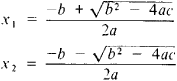# Category Archives: Mathematics

## Math Update Sommer Semester

https://www.mathsisfun.com/calculus/implicit-differentiation.html

https://www.mathway.com/popular-problems/Algebra/200043

## Mental Math Tricks

Will share that soon

## Area of Circle using function

for formula: http://www.mathsisfun.com/area.htm

code:

We have to always remember that value that define is always pass from main function to customized function named by user so here r=3 is passing to function britto and then the britto formula pi*r*r is applying and returned the value and it is printed in the main function where the variable defined as khetrofol.

Here pi declared as global variable with it’s value.

## Find Maximum and Minimum and Summation value from array

Did this problem from subeen vai’s book.

For maximum value:

For minimum value:

Summation:

Average:

Will give here

## LCM – Least Common Multiple

Multiple of 3 is
3×1=3
3×2=6
3×3=9
3×4=12
3×5=15
3×6=18
3×7=21
3×8=24
3×9=27
3×10=30

Multiple of 4 is
4×1=4
4×2=8
4×3=12
4×4=16
4×5=20
4×6=24
4×7=28
4×8=32
4×9=36
4×10=40

Here in 3 and 4 mutilples common is 12
and it is only one value and so it is the lowest also then but if there were some more values than result could be something changed.

So here LCM=12
Here I implemented the code
applied this formula
lcm(a,b)=a*b/gcd(a,b);

code goes here :

## GCD Greatest Common Divisor(Factor)/Highest Common Factor

এখানে লজিকটা হচ্ছে…Here the logic is:
For 12:
1×12=12
2×6=12
3×4=12
So factor of 12=1,2,3,4,6,12
(We don’t need the negative values here)

For 30:
1×30=30
2×15=30
3×10=30
5×6=30
So factor of 30=1,2,3,5,6,10,15,30

Now which is the common factor among 12 and 30
So factor of 12=1,2,3,4,6,12
So factor of 30=1,2,3,5,6,10,15,30

common factor=1,2,3,6
Largest common factor here=6

So now if we divide 12/6 then we get 2
and for 30/6 we get 5

that is all divided perfectly so the logic should be like this

1.Find all the factors of each number
2. Circle the common factors
3. Choose the greatest of those
4. Divide the existing number with greatest common factor thern also get a perfect divide

Here is my code:Another method can also apply here:

Code:

## URI Online Judge Solution| 1036 Bhaskara’s Formula

An equation ax2 + bx + c = 0 where a,b and c are any number  and called the coefficient of the equation
It is known as quadratic equation because any seconbd degree polynomial equation is known as quadratic equation.This equation has two roots x1 and x2This is Bhaskara’s Formula or Bhaskaracharya’s Formula where discriminant D or Del = b24ac
If D>0 then root of quadratic equation are real and unequal
If D=0 then root of quadratic equation are real and equal
If D<0 then root of quadratic equation are conjugate complex number
7s+Formula

So my code is here

## C code using Forward Interpolation

Problem: The population of a town is given below as thousands
Year : 1891 1901 1911 1921 1931
Population : 46 66 81 93 101

Find the population of 1895 ?

Code:

Output:## C code using lagrange method

Problem: The population of a town is given below as thousands
Year            : 1891  1901  1911  1921  1931
Population :  46       66      81      93     101

Find the population of 1895 ?

Code:

Output:## C code using lagrange method

Problem: The population of a town is given below as thousands
Year            : 1891  1901  1911  1921  1931
Population :  46       66      81      93     101

Find the population of 1895 ?

Code:

Output:## C code using lagrange method

Problem: The population of a town is given below as thousands
Year            : 1891  1901  1911  1921  1931
Population :  46       66      81      93     101

Find the population of 1895 ?

Code:

Output:## C code using lagrange method

Problem: The population of a town is given below as thousands
Year            : 1891  1901  1911  1921  1931
Population :  46       66      81      93     101

Find the population of 1895 ?

Code:

Output:## C code using Backward Interpolation

Problem: The population of a town is given below as thousands
Year            : 1891  1901  1911  1921  1931
Population :  46       66      81      93     101

Find the population of 1895 ?

Code:

Output:## C code using Gauss Seidel Method

Problem: Solve the following systems using gauss seidel method
5×1-x2-x3-x4=-4
-x1+10×2-x3-x4=12
-x1-x2+5×3-x4=8
-x1-x2-x3+10×4=34

Code:

Output: